Courses

# Bipolar Junction Transistor MCQ Level -1

## 10 Questions MCQ Test Topic wise Tests for IIT JAM Physics | Bipolar Junction Transistor MCQ Level -1

Description
This mock test of Bipolar Junction Transistor MCQ Level -1 for Physics helps you for every Physics entrance exam. This contains 10 Multiple Choice Questions for Physics Bipolar Junction Transistor MCQ Level -1 (mcq) to study with solutions a complete question bank. The solved questions answers in this Bipolar Junction Transistor MCQ Level -1 quiz give you a good mix of easy questions and tough questions. Physics students definitely take this Bipolar Junction Transistor MCQ Level -1 exercise for a better result in the exam. You can find other Bipolar Junction Transistor MCQ Level -1 extra questions, long questions & short questions for Physics on EduRev as well by searching above.
QUESTION: 1

### Which one of the following is correct for a common base configuration of a transistor?

Solution:

This transistor configuration provides a low input impedance while offering a high output impedance. Although the voltage is high, the current gain is low and the overall power gain is also low

QUESTION: 2

### A pnp transistor can be considered to be equivalent to two diodes as shown in figure.

Solution: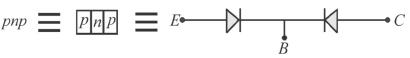The direction of current is always from p to n.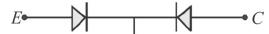QUESTION: 3

### A transistor is used to make a common-base amplifier. If the transistor is npn and when it is biased in the active region, choose the correct option of the following.

Solution:

For an npn common base amplifier, emitter is always forward biased and collector is reverse biased.
The correct answer is: Emitter is forward biased and collector is reverse biased

QUESTION: 4

The frequency response curve of a RC coupled amplifier is shown in the given diagram.Based on it, what is the bandwidth?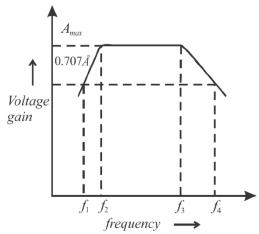Solution:

The difference of frequencies when amplitude becomes 70% of maximum is called as bandwidth.
Hence, bandwidth =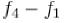The correct answer is:QUESTION: 5

The main function of coupling capacitor in an RC coupled common emitter amplifier is to :

Solution:

The cascaded amplifier, where an RC network is used for inter stage coupling is known as RC coupled amplifier. The main function of capacitor in RC coupling in common emitter amplifier is to isolate the dc .
The correct answer is: dc isolation

QUESTION: 6

The equivalent electrical circuit of a vibrating crystal is as shown below.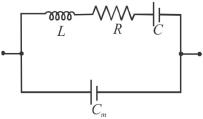Solution:

Since C and Cm are parallel, the equivalent capacitance is given as:
Ceq=c + cm
Now,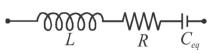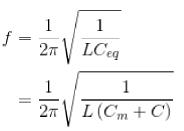The correct answer is: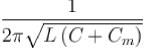QUESTION: 7

In common emitter mode, the input characteristics of a BJT is the variation of :

Solution:

In common emitter mode, lE = lB + lC
The input characteristics of the transistor represents the variation of base current lB with base emitter voltage VBE keeping VCE fixed.
The correct answer is: lB versus VBE at Constant VCE

QUESTION: 8

A crystal oscillator provides a very stable frequency because of.

Solution:

Sharpness is the measurement of the stability. Sharpness increases means durability increases, since sharpness is directly proportional to quality factor Q. Hence, a crystal oscillator provides stable frequency because of high Q of the crystal.
The correct answer is: high Q of the crystal

QUESTION: 9

For a common base circuit configuration of transistor, the correct relation between emitter (lE), base (lB) and collector (lc) current is :

Solution: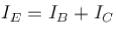The correct answer is: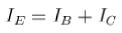QUESTION: 10

The circuit with a common emitter transistor amplifier with conventional stabilized bias is shown in the figure. In this circuit R1 and R2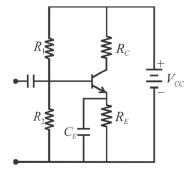Solution:

This is a Voltage divider bias circuit where R1 and R2 along with RE forward bias the base-emitter junction.
The correct answer is: as well as RE forward bias the base-emitter junction